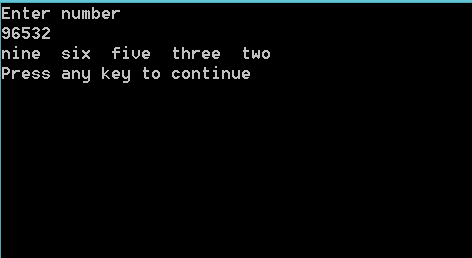## Tuesday, November 26, 2013

### Swap numbers to words>>>>>>>>THE CODE<<<<<<<<

#include<iostream>
#include <math.h>
using namespace std;
void main()
{
int num,i=10,check;
cout<<"Enter number \n";
cin>> num;
//This Part of the program breaks the number into powers
while(true)
{
if((check=num/pow(10,i)) = = 0)
i--;
else break;
}
//This part takes the power and gives the number on that position to the switch
while(num)
{

check= num / pow(10,i);
num= num - check*pow(10,i);
i--;

//This part of the program takes the number and prints the word
switch(check)
{
case 1:
cout<<"one  ";
break;
case 2:
cout<<"two  ";
break;
case 3:
cout<<"three  ";
break;
case 4:
cout<<"four  ";
break;
case 5:
cout<<"five  ";
break;
case 6:
cout<<"six  ";
break;
case 7:
cout<<"seven  ";
break;
case 8:
cout<<"eight  ";
break;
case 9:
cout<<"nine  ";
break;
default:
cout<<"zero  ";

}
}
cout<<endl;

//coded by cppfuzz.com
}